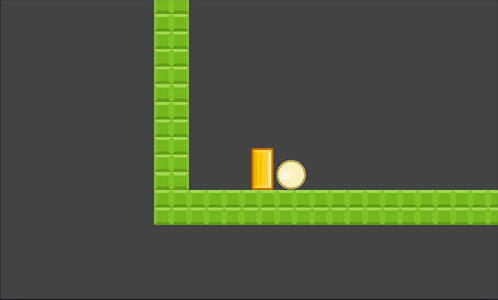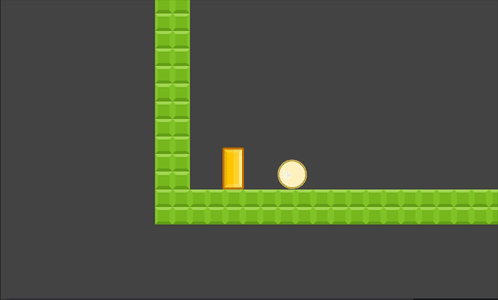# Kinematic to Rigid Body Interaction

## Problem

You want your kinematic character to interact with rigid bodies.

## Solution

By default, a kinematic body moved with `move_and_slide()` or `move_and_collide()` will push any rigid bodies it collides with. This interaction ignores the rigid body’s physics properties due to the kinematic move function’s `infinite_inertia` parameter.In some cases, this might be all you need. However, if you want to avoid “glitches” such as body overlap, tunneling, and other unrealistic behavior, you’ll need to add some code for the interactions.

For this example, we’ll use the 2D character described in the Platform character recipe.

The most commonly used movement method for kinematic bodies is `move_and_slide()`. In the example code, the movement happens on this line:

``````velocity = move_and_slide(velocity, Vector2.UP)
``````

This causes the body to move in the given direction and slide along obstacles when colliding, as well as using the `floor_normal` parameter to determine what surfaces should count as a “floor”. `move_and_slide()` also contains additional parameters:

``````move_and_slide ( Vector2 linear_velocity,
Vector2 floor_normal=Vector2( 0, 0 ),
bool stop_on_slope=false, int max_slides=4,
float floor_max_angle=0.785398,
bool infinite_inertia=true )
``````

It’s that last one that needs to be changed. Since GDScript doesn’t have named parameters, this means we have to pass all of them, but we can keep them at their default values:

``````    velocity = move_and_slide(velocity, Vector2.UP,
false, 4, PI/4, false)
``````

Now if you try to move, you’ll see that the kinematic body just stops on collision. It can’t push the rigid body at all.To give the colliding body a “push” we’ll need to apply an impulse. An impulse is an instantaneous “kick” - think of a bat hitting a ball. This is as opposed to a force, which is a continuous “push” on an object.

``````# This represents the player's inertia.
export (int, 0, 200) var push = 100

func _physics_process(delta):

# after calling move_and_slide()
for index in get_slide_count():
var collision = get_slide_collision(index)
if collision.collider.is_in_group("bodies"):
collision.collider.apply_central_impulse(-collision.normal * push)
``````

The collision normal points out of the rigid body, so we reverse it to point away from the character and apply the `push` factor. Now pushing works again, but it won’t force the rigid bodies through walls:You can also scale the force of the impulse based on the character’s speed:

``````collision.collider.apply_central_impulse(-collision.normal * velocity.length() * push_factor)
# Depending on your character's movement speed, adjust push_factor to
# something between 0 and 1.
``````

Experiment to find the settings that work for your particular game.